﻿ The IMMERSOL model of Radiative Heat Transfer
Encyclopaedia Index
Back to start of radiation-models article

Contents

### 4.1 The main features

#### (a) The dependent variables

IMMERSOL distinguishes in general three temperatures for a control volume in a medium which is tranparent to radiation, namely:
1. T1, the temperature of the first phase e.g. air;
2. T2, the temperature of the second phase, if present, e.g. a cloud of solid paricles suspended within the air; and
3. T3, the 'radiosity temperature' defined below.

If R stands for the radiosity, i.e. the sum all radiation fluxes traversing the volume, regardless of direction and wavelength, then T3 is related to it by the equation:

R = σ T34

where σ is the Stefan-Boltzmann constant, ( = 5.670373x10-8 W m-2 K-4) and T3 is measured in degrees Kelvin, as are the other temperatures. Therefore

T3=(R/σ)1/4    Eq (1)

#### (b) The differential equations

The variables T1 and T2 obey differential equations of the familiar conservation kind. distinguished by special source terms, thus:

∂(c1ρ1T1)/∂t + div(c1ρ1v1 T1) = div(λ1gradT1) + S1,2+ S1,3    Eq (2)

and

∂(c2ρ2T2)/∂t + div(c2ρ2v2 T2) = div(λ2 gradT2) + S2,1+ S2,3    Eq (3)

wherein S1,2 and S2,1 represent energy transfers per unit volume netween phases 1 and 2, and v, the velocity vector. S1,2 and S2,1 volumetric radiative heat absorption and emission. Here the λs represent the thermal conductivities, laminar plus turbulent, of the respective phases. The significance of the other symbols are: c specific heat capacity, ρ density, t time and v velocity vector.

T3 obeys a similar equation but with fewer terms. Specifically it has a zero on the left-hand side, because radiation is not convected In either time or space. It is:

0 = div(λ3 gradT3) + S3,1+ S3,2      (4)

#### (c) The source terms

About S1,2 and S2,1 nothing needs to be said here except that they represent the usual inter-phase transfer processes in the usual way. It is the S1,3, S2,3, S3,1 and S3,2 terms which require discussion.

The IMMERSOL presumption is that they are related to the three temperatures via the following equations:

S1,3 = - S3,1 = 4 ε'1σ(T34-T14)      (5)

and

S2,3 = - S3,2 = 4 ε'2σ(T34-T24)      (6)

wherein ε'1 and ε'2 are the emissivities of the respective phases per unit length. These quantities are supposed numerically equal to the absorptivities, which measure the proportion of the radiation flux which is absorbed per meter of its passage through the medium in question.

#### (d) The value ascribed to λ3

IMMERSOL expresses the thermal conductivity pertaining to the radiosity temperature T3, in terms of: the emissivities per unit length, ε'and the scattering coefficients per unit length, s' of the two phases in the transparent-to-radiation space; and the gap between nearby solid walls', Wgap as:

λ3 = 4 σT33/ { 0.75( ε'1 + s'1 + ε'2 + s'2) + 1/Wgap }      (7)

#### (e) The boundary conditions

At the walls of, and everywhere within, solid bodies surounding or immersed in the transparent medium, T3 is taken as being equal to the temperature T1 or T2, according to the phase in question. However the radiant flux at such walls depends not only on the T3-gradient in the medium close to the wall. but also on the surface emissivity of the wall itself, in a manner which will be discussed in section 4.2 (d) below.

At open boundaries of the domain, nett radiation fluxes are ordinarily prescribed.

### 4.2 The rationale

#### (a) Starting points

In its neglect of wave-length dependency, the IMMERSOL model departs radically from reality; but it does so in a manner which is commnonly regarded as acceptable: it employs the widely-used 'grey-medium' approximation described in many text-books. for example in Sparrow and Cess (1966), Chapter 7.

In that book, and elsewhere, two other accepted ideas are described which IMMERSOL has incoporated, namely those of the 'optically-thick' and 'optically-thin' limits. Here 'thick' and 'thin' compare the size of the gap between the solid walls enclosing the transparent medium with what can be called the 'mean free path' of radiation, i.e. the reciprocal of ε'+s'.

What is distinctive about IMMERSOL is that it is valid both for and between those limits.

Both extremes arise in practice. Within a large coal-fired furnace, the cloud of burning particles and gaseous combustion products can be regarded as optically thick; for so much solid surface is present per unit volume that rays emanating from the middle of the furnace must be multiply reflected before they escape to the water-cooled walls. The air within the rooms and corridors of inhabited buildings, by contrast, constitutes an optically thin medium; wall-to-wall radiation suffers no impediment.

For optically thick media, there exists a formula which connects the radiative heat flux q, in W/m2, with the gradient of the radiosity. It is usually associated with the name of Rosseland; and it is:

q = -(4/3)(ε'+s')-1 σ grad(T4)      (8)

Here T is the local temperature of the transparent medium.

If the equation is expressed in terms of an effective thermal conductivity, λeff, involving grad T rather than grad(T4), the expression for λref becomes:

λeff = (16/3) (ε'+s')-1 σ T3      (9)

At the other extreme, when the medium is so thin as not to participate at all in the radiative heat transfer between two solid sufaces, at temperatures T hot and Tcold, say, the heat flux q is well known to obey the formula:

q ={1 + (1-εhot)/εhot + (1-εcold)/εcold }-1σ(Thot4 - Tcold4)
={1/εhot + 1/εcold - 1}-1σ(Thot4 - Tcold4)      (10)

Equation (8) is of the flux-proportional-to-gradient kind which PHOENICS is well-equipped to solve. Equation (10) is of the less amenable action-at-a distance kind. The question arises how can the latter be made more like the former?

#### (b) First steps

Re-writing equation (10) for the case in which (Thot - Tcold) is small and in which the wall emissivities are unity, yields:

q = 4σT3(Thot-Tcold)      (11)

where T stands for either temperature

Since the temperature gradient equals (Thot - Tcold)/Wgap, the effective conductivity which corresponds to equation (11) is simply:

λeff = 4WgapσT3      (12)

where Wgap stands for the distance between the solid surfaces. So the conductivity increases with inter-wall distance; as it must do if the heat flux is to be independent of that distance.

It is interesting to compare the value of this conductivity with the thermal conductivities of common materials such as:

 atmospheric air water at 0 degC steel 0.0258 0.569 43.0
wherein the units are W m1 degC1.

In the same units, and with a wall gap equal to one metre, the values of λeff at various temperatures in degrees Celsius are:

 T3 20 100 500 1000 1500 2000 λeff 5.706 11.77 104.8 467.9 1264.1 2663.6
Even taking into account that turbulence may increase the effective conductivity of a fluid by two or three orders of magnitude, it can be concluded from these tables that radiative heat transfer can be significant at room temperature; and at high temperatures it becomes dominant.

#### (c) Between the 'thick' and 'thin' extremes.

Let now the reciprocal of conductivity be considered, i.e. the resistivity, measured in degC m / W. For the thick medium eq.(9) yields:

λeff-1 = (3/16)(ε'+s')/(σ T3)      (13)

and, for the thinnest-possible totally-empty medium, eq. (12) yields:

λeff-1 = 1/(4Wgapσ T3)      (14)

It is therefore not unreasonable to suppose that, for intermediate conditions, the two multipliers of 4σ T3 should be added so as to create a more-generally-valid single resistivity formula thus:

λeff-1 = { (3/4) (ε'+s') + 1/Wgap }/(4σ T3)      (15)

This is the origin of equation (7), introduced in section 4.1(d) above.

#### (d) Wall emissivity as an extra resistance

Equation (7) is used for the calculation of the T3-diffusion fluxes of the finite-volume equations which PHOENICS solves; but something special is done for coefficients when one node lies in the transparent medium and the other within a solid, as exemplified by nodes B and A in the following figure, in which, for simplicity, the transparent medium consists of a single phase: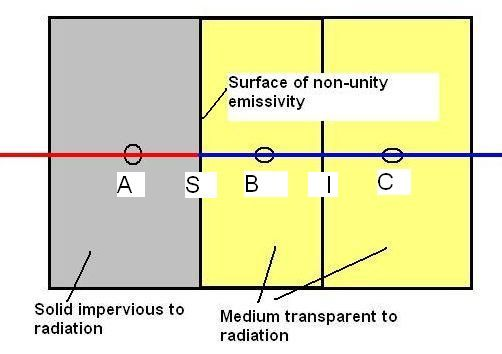As is usual in PHOENICS, the conductivities pertaining to the cell are stored at each grid node; Therefore the radiative heat flux qr,B-C crossing the boundary between cell B and cell C will be deduced from the formula:

qr,B-C = (T3,B - T3,C)/{(xI-xB)/λ3,B + (xC-xI)/λ3,C)}     Eqn (15a)

where x is the horizontal co-ordinate and the λ's are given by equation (15) above.

However, the calculation of the radiant flux at the S interface requires more careful study because the surface emissivity can cause a discontinuity of T3 gradient there, as is illustrated in the following figure, which shows the postulated profiles of both T3 and T1 because of their inescapable interaction.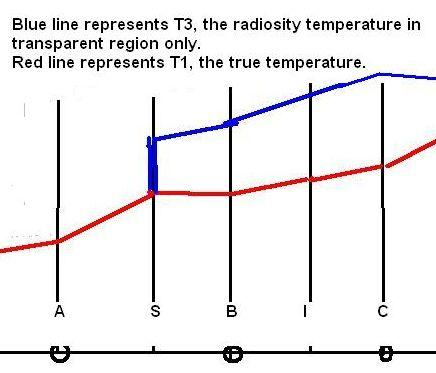Here it is postulated that T3 and T1 are equal to each other within the solid but, whereas the latter has a finite gradient everywhere, the latter may have an infinite one at the interface between the phases.

The fluxes of energy in question are as follows:

• conduction from A to S, namely:

qA-S = (T1,A-T1,S)/RA-S    Eqn 15(b)
RA-S = (xS-xA)/λ1,A     Eqn 15(c)

• conduction and convection from S to B, namely:

qc,S-B = (T1,S-T1,B)/Rc,S-B    Eqn 15(d)
Rc,S-B = (xB-xS)/λ1,B     Eqn 15(e)

• radiation from S to B, namely:

qr,S-B = (T3,S-T3,B)/Rr,S-B     Eqn 15(f)
Rr,S-B = (xB-xS)/λ3,B+(1-εS)/[εS4σT33]    Eqn 15(g)

wherein the term involving εS is inserted so as to conform with equation (10) above.

Requiring them to be in balance at the surface S enables the equal-by-definition values of T3 and T1 here to be evaluated. Specifically, the heat balance at the surface S is given by:

qA-S = qc,S-B + qr,S-B     Eqn 15(h)

and with T1S=T3S, the necessary formula can be deduced from eqn 15(h) as follows:

T1,S = T3,S = (T1,A/RA-S + T1,B/Rc,S-B + T3,B/Rr,S-B)/(1/RA-S+1/Rc,S-B+1/Rr,S-B)     Eqn(16)

where the resistances RA-S, Rc,S-B and Rr,S-B were defined by equations 15(c), 15(e) and 15(g) earlier. Inspection of the Rr,S-B term in equation 15(g) shows that (xB-xS)/λ3,B is the conventional resistance and that (1-εS)/(εS4σT33) is the extra resistance caused by the non-unity emissivity.

#### (e) The radiative heat flux at a wall

From equations 15, 15(f) and 15(g) above, the radiative heat flux at the wall can now be written as:

qr,S-B = ΓS-B(T3,S-T3,B)     Eqn (17)

where

ΓS-B = 4σT3,m3/[(xB-xS)(0.75(ε'+s')+1/Wgap) + (1-εw)/εw]    Eqn (18)

and T3,m is a mean temperature between S and B, which is evaluated from:

T3,m3=(TS2+TB2)(TS+TB)/4     Eqn (19)

This expression follows from linearising the wall heat flux, as follows:

qr,S-B = σβ(TS4-TB4) = σβ(TS2+TB2)(TS2-TB2) qr,S-B = 4σβT3,m3(TS-TB)    Eqn (20)

where by comparison with equation (18) above it is clear β is defined by:

β = [(xB-xS)(0.75(ε'+s')+1/Wgap) + (1-εw)/εw]-1     Eqn (21)

### (a) Activation via Q1

Few actions are needed in order to activate IMMERSOL. They comprise:

SOLVE(T3) to ensure that T3 is solved

DISWAL to activate the WDIS and WGAP calculation

STORE(WGAP) to enable this quantity to be accessed

together with such information as provides material properties and initial and boundary conditions.

#### Warning

It is also desirable to set varmax(tem1) and varmax(t3) to the lowest reasonable value, lest unrealistic temperatures generated at the start of iteration fail, because of the inherent non-linearity of the equation system, to die away.

Examples may be found in the IMMERSOL-related cases, in the advanced-radiation-option library.

### (b) Setting emissivities/absorptivities and scattering coefficients

When it was first created, IMMERSOL was provided with means of supplying relevant property data by way of the PIL variables RADIA, RADIB, and of special SPEDAT statements,

From PHOENICS-3.4 onwards, however, the setting of radiation-related properties has been made more similar to the setting of other properties, such as density and specific heat.

Thus:

• there now exist two new PIL variables with meaningful names, namely:
EMISS,
the emission (and absorption) coefficient of a transparent medium, with the dimensions of 1/length; and
SCATT,
the scattering coefficient of the transparent medium, also with the dimensions of 1/length

• If these quantities are set to non-negative values, those values are used for all regions which are not occupied by solids, unless further instructions are given.

• Negative values are treated as zero, being without either physical or formula-choosing significance[ unlike the case of RHO1, etc].

• If the commands:
STORE(EMIS) and/or STORE(SCAT)
are placed in the Q1, for print-out or other purposes, the fields of values are printed in the result file, and stored in the phi file, in the usual manner.

Moreover different values can then be placed in different parts of the domain by the conventional use of:
FIINIT( ),
PATCH(name,INIVAL, ),
INIT(name, EMIS, ) and
INIT(name, SCAT, )

• If InForm-style property formulae are the provided in the Q1, it is the values which the formulae provide which are used in the radiation calculations.

• PLANT-generated or user-generated Fortran coding can also be used to provide non-uniform emissivity and scattering coefficients.

The differences from the treatment of other properties are:

• no entries have been provided for emissivity and scattering coefficients in the PROPS file, because it is usually small contaminants ot the pure materials which affect the radiation, for example water droplets (mist) in air, or soot particles in combustion gases; and

• no GX-style formulae, accessed via GRNDx settings, are provided, because the availability of InForm.

Examples will be found in the input-library cases:
r201.htm, r202.htm, r203.htm and r204.htm. However, it should be noted that, when EMIS is set for a cell containing a non-transparent solid, it becomes dimensionless; and it then represents the emissivity/absorptivity of the surface of the solid.

### (c) Domain-boundary conditions

Although the settings of EMIS within the transparent and non-transparent media suffice to enable internal-to-the-domain boundaries to be correctly represented, there is also sometimes a need to ascribe radiation-influencing conditions at the boundaries of the domain.

For example, it may be desired to represent an aperture in the bounding surface, though which radiation can enter and leave; or a domain boundary may be required to simulate a solid wall of prescribed temperature, for which the emissivity must also be defined.

To meet this requirement, PHOENICS has been caused to react appropriately to PATCH and COVAL statements, for which the patch-name begins with the characters 'IM' and the dependent variable in question is T3.

How such patches are used is explained in the section of the lecture on IMMERSOL which may be viewed by clicking here,

### (d) Wall-boundary conditions

At a wall boundary of known temperature and emissivity the following boundary conditions are applied:

(a) PLATE

For a specified wall temperature and emissivity, the radiative heat flux at the wall is computed from:

qw = σβ(Tw4-T3,p4) = 4σβT3,m3(Tw-T3,p)    Eqn (22)

where the wall resistance term β is given by:

β = [δ(0.75(ε'+s')+1/Wgap) + (1-εw)/εw]-1     Eqn (23)

and T3,m is a mean temperature between wall w and the near-wall node p, which is evaluated from:

T3,m3=(Tw2+T3,p2)(Tw+T3,p)/4     Eqn (24)

(b) Participating BLOCKAGE

Likewise, at the solid-fluid interface of a participating BLOCKAGE, the radiative heat flux at the wall for a specified emissivity is computed from:

qw = σβ(Ts4-T3,g4) = 4σβT3,m3(Ts-T3,g)    Eqn (25)

where the wall resistance term β is given by:

β = [δ(0.75(ε'+s')+1/Wgap) + (1-εw)/εw]-1     Eqn (26)

and T3,m is a mean temperature between solid node and the fluid node g, which is evaluated from:

T3,m3=(Ts2+T3,g2)(Ts+T3,g)/4     Eqn (27)

(c) Non-participating BLOCKAGE

In PHOENICS 2018 and earlier, no action is taken at the adiabatic surface so that both the convective and radiative heat flux are zero.

In PHOENICS 2019 and onwards, both the radiative and convective heat flux are evaluated at the surface, and surface temperature is computed from the requirement that the total heat transfer rate is zero.

(d) THIN PLATE

The boundary conditions applied on either side of a thin plate are explained in detail here

### 4.5 An example: Input-file library case r209

The application of IMMERSOL to a steady-state heating-and-cooling problem will be described, by reference to the following sketch:#### Problem-specifying input data

• The flow is steady and laminar.
• The Reynolds number is 10
• The pressure is atmospheric.
• The dimensions are small and the air is unpolluted, so that it does not participate at all in the radiative process.
• The emissivities of the surfaces are:-
• bottom surface... 0.9
• top surface.... 0.9
• first solid...... 0.1
• second solid... 0.2

The practically interesting question is: What temperatures will be attained in the solid?

First some images showing the geometry and some results: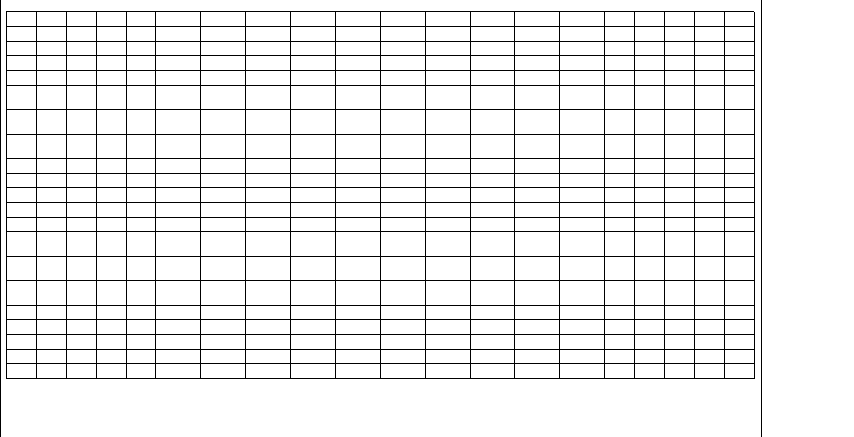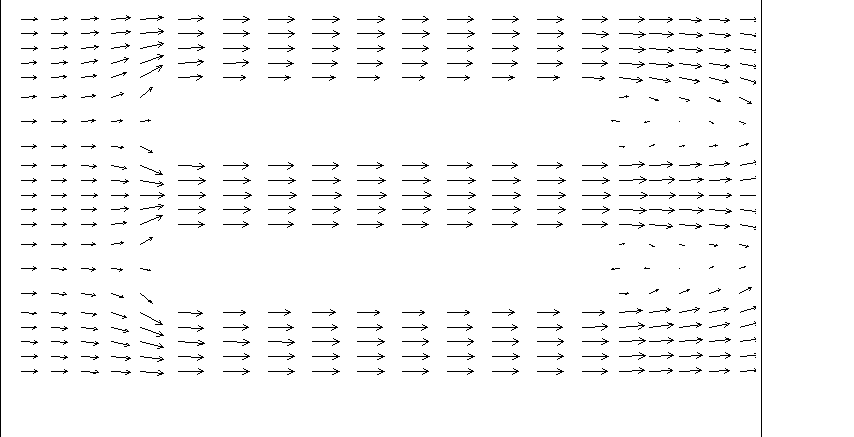The grid and velocity vectors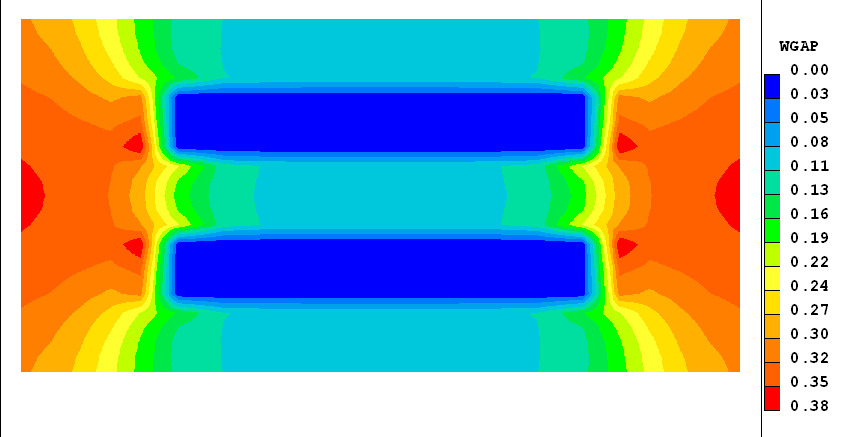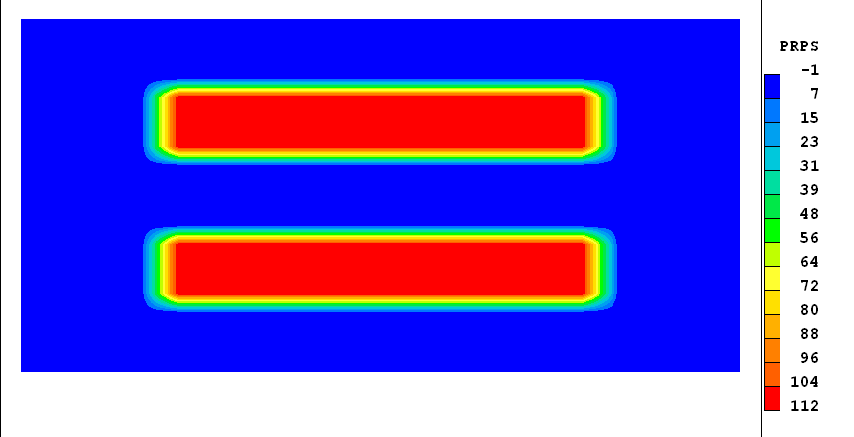The distributions of WGAP and of PRPS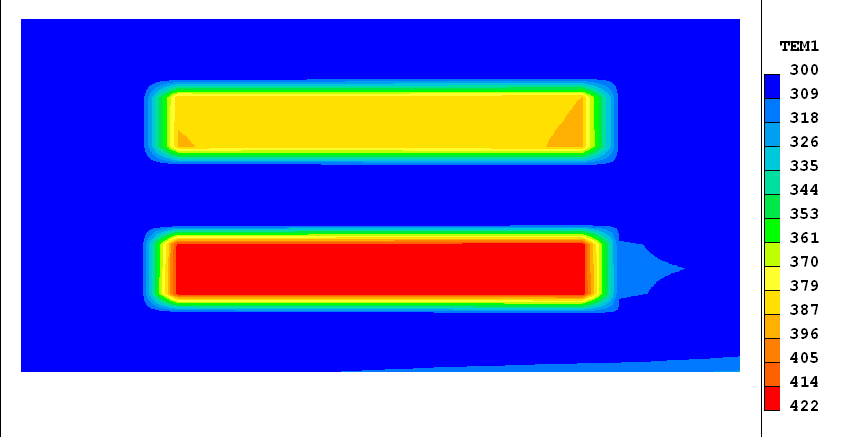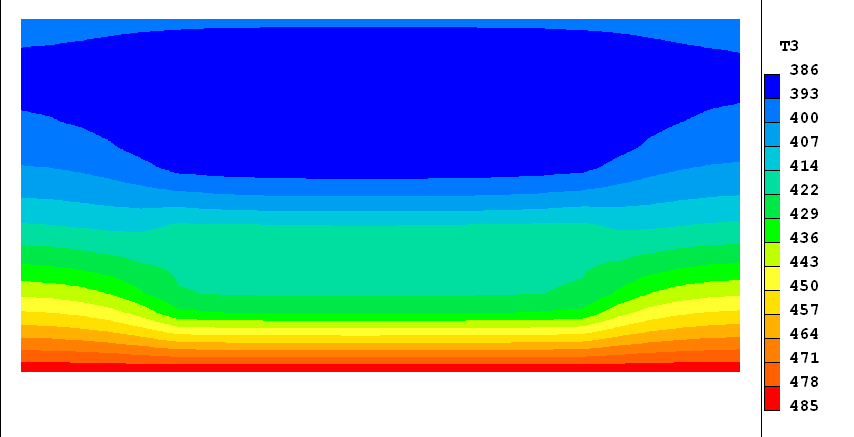The true (TEM1) and radiation (T3) temperatures

Evidently both plates are heated, by radiation, to temperatures significantly above that of the cooling air which surrounds them.

Since it is not easily seen from the above contour plot that TEM1 and T3 are very close within the solid, the following plot is provided of the difference between them. This is easily calculated by placing the following In-Form line in the Q1 file.

`(stored tdif is tem1-t3)`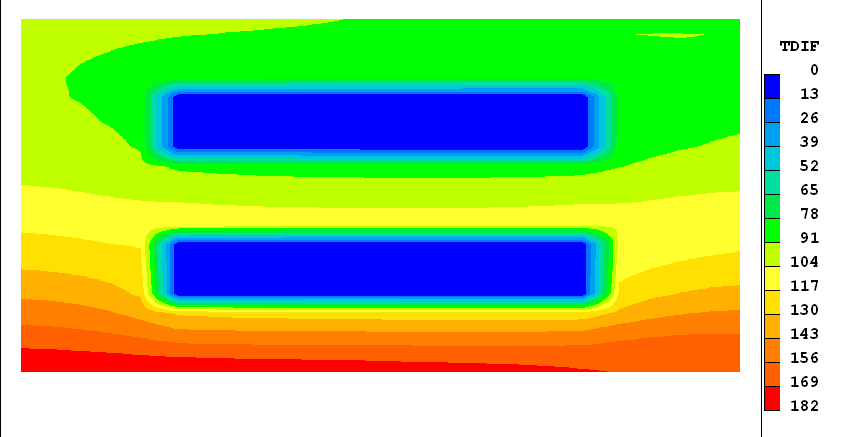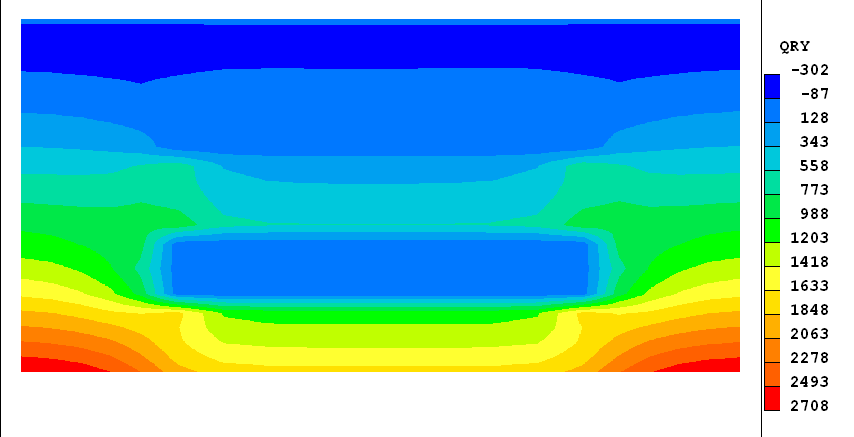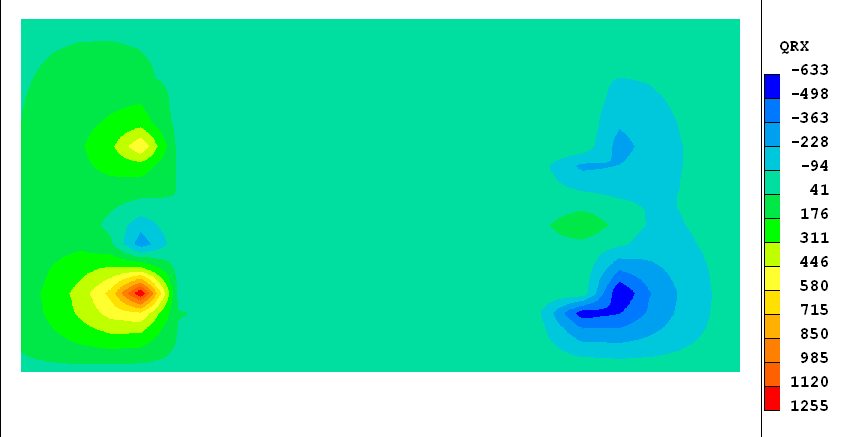The upward (y) and rightward (x) radiative heat fluxes

The y-direction fluxes are the greater, because it is that direction that the temperature variations are largest and both negative (downward directed) and positive (upward-directed) fluxes are in evidence.

IMMERSOL has allowed this problem to be set up and solved with great ease, the advantage of which can be best appreciated by those who have attempted to solve such problems by the methods advocated in many text-books, involving use of 'view-factor' calculations.

### 4.7 Concluding remarks regarding IMMERSOL

• IMMERSOL was conceived and introduced into PHOENICS during 1996.

• Wherever tested, it has performed well, in respect of:
• accuracy, where exact solutions are known;
• plausibility, where they are not;
• economy, in all circumstances.

• Since it is the ONLY model which can be economically used in complex circumstances, including multi-phase, chemically reactive and body-fitted coordinates, it seems likely to become the most widely used of all the radiation models in PHOENICS.

### 4.8 References

1. Spalding, D.B: Trends, Tricks and Try-ons in CFD/CHT. In Advances in Heat Transfer, Vol.45, pp1-78, Editors: E.M.Sparrow, Y.I.Chou, J.P.Abraham, J.M.Gorman, Academic Press, Elsevier. (2013)

End of the IMMERSOL section

Previous

Next